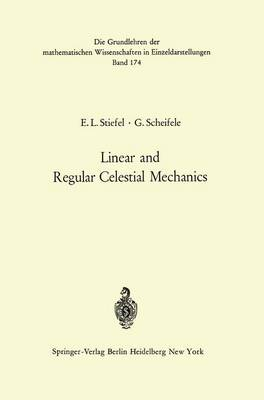Days : Hours : Minutes : Seconds•# Linear and Regular Celestial Mechanics: Perturbed Two-Body Motion Numerical Methods Canonical Theory - Grundlehren der Mathematischen Wissenschaften 174 (Hardback)

(author), (author)
£72.00
Hardback 316 Pages / Published: 01/01/1971
• Not available

This product is currently unavailable

This product is currently unavailable.

Our presentation of some fundamental domains of celestial mechanics requires no special preliminary knowledge; however, the chosen mathe- matical method is new in so far as the pure two-body motion is described by linear differential equations, which even have constant coefficients. In other words an equivalence between the Keplerian motion and a harmonic oscillation is established; this approach to celestial mechanics will be referred to as the linear theory. Besides the possibility of the mutual fruitful interaction between celestial and oscillatory mechanics which is thereby created, our linear differential equations are as a result everywhere regular. The opposite is true of the classical Newtonian equations, which are singular at the collision of the two moving bodies"'Reg~larization is however not the leitmotiv of the book; the many regularization methods  which do not lead to linear differential equations are therefore not described. Apart from the basic idea of the use of linear differential equations and the resulting advantages, there were two further scientific goals which we had in mind. First, it should be permissible not only to transform the coordinates of the mobile but also to introduce other independent variables instead of the time. The often cumbersome solution of the Keplerian equation in theoretical studies can thereby be avoided. This leads to the further consequence that the linear theory is uniform with respect to the value of the eccentricity.

Publisher: Springer-Verlag Berlin and Heidelberg GmbH & Co. KG
ISBN: 9783540051190
Number of pages: 316
Weight: 550 g